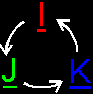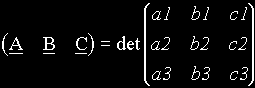3D Geometry Primer: Chapter 1 - Issue 06 - New Light On Vector Arithmetic by (18 September 2000) Return to The Archives
 New Light On Vector ArithmeticWe already know how to represent vectors by values. It would be nice if we could do all vector arithmetic using those values. And that's exactly what we're going to find this week.We're going to do very *heavy* math here. As you can see, there are no pictures this week (except for two). Everything will be pure math! For those people who are petrified by this, read this anyway but skip the parts that are indicated with "prove" ;-)How are we going to do this? We know that each vector is a linear combination of the 3 unit vectors. e.g. A(a1,a2,a3) = a1*I + a2*J + a3*K. That fact is the key to translate all vector operations to real numerical operations. For each operation, the first step will be writing each vector as a linear combination of the unit vectors. At that moment there will be only real values and unit vectors left, We'll work that out till we get back a linear combination of exactly 1 I vector, 1 J vector and 1 K vector.We'll use vectors A(a1,a2,a3), B(b1,b2,b3) and C(c1,c2,c3) as input vectors.. The vector R(x,y,z) will be the result of the vector operation.One more thing before we start. I've gathered somekind of "knowledge base" about vectors in one file. It contains most of the properties of vector arithmetic, you need today. It also already contains the new stuff we'll learn today and some other properties not mentioned in this Primer at all. Those other properties are there without further explonation, but you can use them as they are, *if* you will ever need them. You can download it here.

 (I) Negative Of A Vector: –ATo get the negative of a vector A(a1,a2,a3), just take the negative of each component value: R(x,y,z) = –A(a1,a2,a3) = R(–a1,–a2,–a3) Thus: x=–a1, y=–a2 and z=–a3 e.g.: –U(1,2,3)=R(–1,–2,–3) PROVE: –A(a1,a2,a3) = –(a1*I + a2*J + a3*K) use t*(r*U+s*V) = (t*r)*U+(t*s)*V = (–a1)*I + (–a2)*J + (–a3)*K = R(–a1,–a2,–a3)

 (II) Scalar Product: r*ATo multiply a vector A(a1,a2,a3) with a scalar r, just multiply the x-,y- and z-components with this scalar R(x,y,z) = r*A(a1,a2,a3) = R(r*a1, r*a2, r*a3) Thus: x=r*a1, y=r*a2, z=r*a3 e.g.: 2*U(1,2,3) = R(2*1, 2*2, 2*3) = R(2,4,6) PROVE: r*A(a1,a2,a3) = r*(a1*I + a2*J + a3*K) use t*(r*U+s*V) = (t*r)*U+(t*s)*V = (r*a1)*I + (r*a2)*J + (r*a3)*K = R(r*a1,r*a2,r*a3)

 (III) Vector Addition: A+BTo add 2 vectors together, add the respective components together R(x,y,z) = A(a1,a2,a3) + B(b1,b2,b3) = R(a1+b1, a2+b2, a3+b3) Thus: x=a1+b1, y=a2+b2, z=a3+b3 e.g.: U(1,2,3)+V(4,5,6) = R(1+4, 2+5, 3+6) = R(5,7,9) PROVE: A(a1,a2,a3) + B(b1,b2,b3) = (a1*I + a2*J + a3*K) + (b1*I + b2*J + b3*K) use r*V+s*V = (r+s)*V = (a1+b1)*I + (a2+b2)*J + (a3+b3)*K = R(a1+b1, a2+b2, a3+b3) Same thing for more vectors: A(a1,a2,a3) + B(b1,b2,b3) + C(c1,c2,c3) = R(a1+b1+c1, a2+b2+c2, a3+b3+c3)

 (IV) Vector Subtraction: A–BTo subtract 2 vectors, subtract the respective components R(x,y,z) = A(a1,a2,a3) – B(b1,b2,b3) = R(a1–b1, a2–b2, a3–b3) Thus: x=a1–b1, y=a2–b2, z=a3–b3 e.g.: U(1,2,3)–V(4,5,6) = R(1–4, 2–5, 3–6) = R(–3,–3,–3) PROVE: Take a look at (i) and (iii) and try it by yourself.

 (V) Dot Product: A·BThe dot product of 2 vectors A(a1,a2,a3) and B(b1,b2,b3) is given by the next identity: A·B = a1*b1 + a2*b2 + a3*b3 e.g.: U(1,2,3)·V(4,5,6) = 1*4 + 2*5 + 3*6 = 32 PROVE: A(a1,a2,a3)·B(b1,b2,b3) = (a1*I + a2*J + a3*K)·(b1*I + b2*J + b3*K) use (r*U+s*V)·(t*W+x*Z) = (r*U)·(t*W+x*Z)+(s*V)·(t *W+x*Z) = (a1*I)·(b1*I + b2*J + b3*K) + (a2*J)·(b1*I + b2*J + b3*K) + (a3*K)·(b1*I + b2*J + b3*K) Now, we'll work out the first term (a1*I)·(b1*I + b2*J + b3*K) = (a1*I)·(b1*I) + (a1*I)·(b2*J) + (a1*I)·(b3*K) use (r*U)·(s*V)=(r*s)*(U·V) = (a1*b1)*(I·I) + (a1*b2)*(I·J) + (a1*b3)*(I·K) we know that I, J and K are perpendicular to each other => I·J=0, I·K=0 and J·K=0 (zero) = (a1*b1)*(I·I) we know that I is normalized (it has magnitude 1) and the angle "between I and I" is 0 (zero) => I·I=1 = a1*b1 Same story for the 2nd and the 3rd term. You'll get a2*b2 and a3*b3 respective. So at the end we get: = a1*b1 + a2*b2 + a3*b3 NOTICE THAT: ° You can use this to calculate the magnitude of a vector A because ||A||˛ = A˛. Thus ||A|| = sqrt(A·A) = sqrt(a1*a1+a2*a2+a3*a3) ° You can use this too to calculate the angle between 2 vectors. Compare the new definition with the old one: A·B = ||A||*||B||*cos(theta) = a1*b1+a2*b2+a3*b3. Now seperate cos(theta) and you get: cos(theta) = (a1*b1+a2*b2+a3*b3) / (||A||*||B||). You can calculate the magnitudes ||A|| and ||B|| already, thus you can calculate cos(theta) now. ° Try to avoid the use of the square root. Sometimes it is possible to do the same thing without it. e.g. you need to check if the magnitude of a vector A is smaller that a certain value r. You can do that with the question "sqrt(A·A) < r ?". But you can also ask the same thing with "A·A < r*r ?". This is much faster and it gives the same result.

 (VI) Cross Product: A×BThe cross product of 2 vectors A(a1,a2,a3) and B(b1,b2,b3) is again a vector R(x,y,z). To find this vector you need to calculate the 3 components x, y and z. Those components are given by next identities: x = a2*b3 – a3*b2 y = a3*b1 – a1*b3 z = a1*b2 – a2*b1 Notice that the indices are cyclic increased each line: x->y->z->x->...; a1->a2->a3->a1->...; b1->b2->b3->b1->...e.g.: U(1,2,3)×V(4,5,6)=R(2*6–3*5, 3*4–1*6, 1*5–2*4) = R(–3, 6, –3) PROVE: This is much similar to the dot product A(a1,a2,a3)×B(b1,b2,b3) = (a1*I + a2*J + a3*K)×(b1*I + b2*J + b3*K) use (r*U+s*V)×(t*W+x*Z) = (r*U)×(t*W+x*Z)+(s*V)×(t *W+x*Z) = (a1*I)×(b1*I + b2*J + b3*K) + (a2*J)×(b1*I + b2*J + b3*K) + (a3*K)×(b1*I + b2*J + b3*K) Now, we'll work out the first term (a1*I)×(b1*I + b2*J + b3*K) = (a1*I)×(b1*I) + (a1*I)×(b2*J) + (a1*I)×(b3*K) use (r*U)×(s*V)=(r*s)*(U×V) = (a1*b1)*(I×I) + (a1*b2)*(I×J) + (a1*b3)*(I×K) we know that I×I=0, J×J=0, K×K=0; and that I×K=–(K×I), J×I=–(I×J), K×J=–(J×K) = (a1*b2)*(I×J) + (a1*b3)*–(K×I) we know that I×J=K, J×K=I and K×I=J = (a1*b2)*K – (a1*b3)*J Same story for the 2nd and the 3rd term. You'll get (a2*b3)*I – (a2*b1)*K and (a3*b1)*J – (a3*b2)*I respective If we put all things together, then we get: = (a1*b2)*K – (a1*b3)*J + (a2*b3)*I – (a2*b1)*K + (a3*b1)*J – (a3*b2)*I and after regrouping... = (a2*b3 – a3*b2)*I + (a3*b1 – a1*b3)*J + (a1*b2 – a2*b1)*K

 (VII) Mixed Product: (A B C) = A·(B×C)Now you know how to calculate a dot product and a cross product, so you can calculate this mixed product. Here it is in one step:(A B C) = a1*(b2*c3–b3*c2) + a2*(b3*c1–b1*c3) + a3*(b1*c3–b3*c1)Of course, this is an awful to remember. Fortunatelly, this is exactly the same as solving the following determinant (remark 1)That's all for today. You should know enough now, to use vectors in your programs. You can store them, and you can do arithmetic with them. That's all you need. I've gathered some information in a kind of knowledge base. You should find all of the information you need in it to solve vector problems. In it, there are some new identities that aren't discussed here, but you can use them literally like they are mentioned there. If you don't know what det() means, just read the stuff beside it. It works fine too. (remark 1)Next week, we're going to try to extend vectors to points.If you want a little exercise, try this: I give you these 5 vectors: A(1,0,1), B(0,1,1), C(2,0,–4), D(3,2,1) and E(1,1.5,2). Solve the following expressions (numerical): R(x,y,z) = (A+2*B) × ((C·D)*E) S(x,y,z) = (A×(–B)) · (–C+D+E) It shouldn't be too hard to solve it by hand, but remember to work out the brackets first!Regards, Bramz

 Remarks(1) Don't panic if you don't understand anything about matrices. You can still do the job without it. Just ignore them, if you don't like them. However, if you want to know more about matrices, check these out: "Matrices: an introduction" by Lionel Brits (http://www.cadvision.com/britsc/tgc/tutorials/matrices.htm) "Matrix operations" by Frenzy (http://www.foxfiber.net/frenzy/matrix.htm) "Matrix and Quaternion FAQ" by hexapod@netcom.com (http://www.flipcode.com/documents/matrfaq.html)